## Welcome to Convert.net

### Convert.net can evaluate mathematical expressions involving basic arithmetic, advanced math and logic, and conversions of a large number of unit measurements in different fields.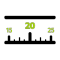Length

In geometric measurements, length most commonly refers to the measurement of one dimension of an object.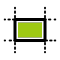Area

Area is a quantity that expresses the extent of a two-dimensional surface or shape, or planar lamina, in the plane.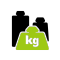Weight

In science and engineering, the weight of an object is usually taken to be the force on the object due to gravity.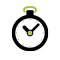Time

Time is a dimension in which events can be ordered from the past through the present into the future.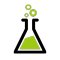Volume

Volume is the quantity of three-dimensional space enclosed by some closed boundary, for example, the space that a shape contains.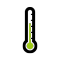Temperature

Temperature is a physical property of matter that quantitatively expresses the common notions of hot and cold.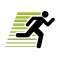Velocity

Velocity is the rate of change of the position of an object, equivalent to a specification of its speed and direction of motion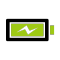Power

Power is the rate at which energy is transferred, used, or transformed. E.g. the rate at which a light bulb transforms electrical energy into heat.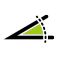Angles

An angle is the figure formed by two rays, called the sides of the angle, sharing a common endpoint, called the vertex of the angle.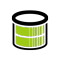Data Storage

Memory, components, devices and media that retain digital computer data used for computing for some interval of time.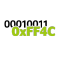Number Systems

A number system is a way of counting things. It's a way of identifying the quantity of something.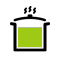Cooking

In recipes, quantities of ingredients may be specified by mass (commonly called weight), by volume, or by count.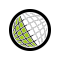Time Zones

A time zone is a region on Earth that has a uniform standard time for legal, commercial, and social purposes.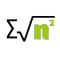Math

Mathematics is the abstract study of topics encompassing quantity, structure, space, change, and other properties.